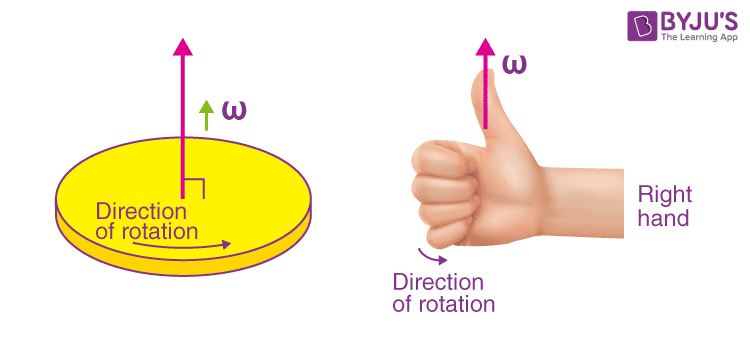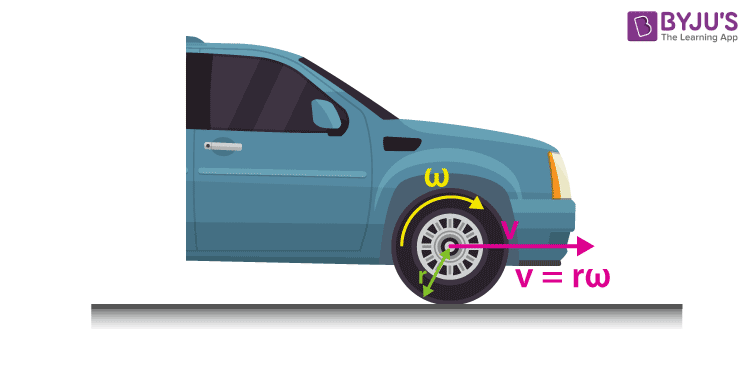# Angular Velocity

Angular velocity is an important physics concept that is applied to objects that move along a circular path. This article will explore the concept of angular velocity and its relationship with linear velocity.

## What is Angular Velocity?

In this section, you will understand angular velocity and its role in rotational motion.

To understand angular velocity, you need to understand what rotation is. To help you with your understanding, let us consider a windmill. A windmill is a rigid body that rotates about a fixed axis. A windmill exhibits rotational motion when the blades of the windmill rotate around an axis that passes through the rotor. The velocity associated with rigid bodies as they exhibit rotation about a fixed axis is called angular velocity.

### Angular Velocity Definition

In physics, we define angular velocity as follows:

Angular velocity is the vector measure of the rotation rate, which refers to how fast an object rotates or revolves relative to another point.

In simple words, angular velocity is the time rate at which an object rotates or revolves about an axis. Angular velocity is represented by the Greek letter omega (ω, sometimes Ω). It is measured in angle per unit time; hence, the SI unit of angular velocity is radians per second. The dimensional formula of angular velocity is [M0 L0 T-1].

For an object rotating about an axis, every point on the object has the same angular velocity. But points farther from the axis of rotation move at a different tangential velocity than points closer to the axis of rotation. Angular velocity is also known as rotational velocity and angular frequency vector.

Watch the video and understand the significance of angular velocity in detail.## Angular Velocity Formula

Since the angular velocity of an object is the object’s angular displacement with respect to time, angular velocity is expressed as follows:

$$\begin{array}{l}\omega =\frac{\Theta }{t}\end{array}$$

Where ω is the angular velocity, θ is the angular displacement, and t is the change in time t.

By convention, positive angular velocity indicates counterclockwise rotation, while negative is clockwise.

### Average Angular Velocity

The average angular velocity of a rotating rigid body is the ratio of the angular displacement to the time interval.

$$\begin{array}{l}\omega _{avg}=\frac{\Delta \Theta }{\Delta t}=\frac{\Theta_{2}-\Theta_{1} }{t_{1}-t_{2}}\end{array}$$

### Instantaneous Angular Velocity

The instantaneous angular velocity is defined as the limit of the average angular velocity as the time interval approaches zero.

$$\begin{array}{l}\omega_{ins}=\lim_{\Delta t\rightarrow 0}\frac{\Delta \Theta }{\Delta t}=\frac{d\Theta }{dt}\end{array}$$

## Finding the Angular Velocity Direction

The angular velocity direction is difficult to track because a point on a rotating object constantly changes direction. The axis of the rotating object is the only point where the object has a fixed direction. With the help of the axis of rotation, the direction of angular velocity is determined by the Right Hand Rule.

### Right Hand Rule

The direction of angular velocity is found by using the right hand rule. For better understanding, consider a spinning disc, as shown in the figure below. Imagine a pole passing through the centre of the disc at the axis of rotation. Using the right hand rule, your right hand would be grasping the pole so that your four fingers follow the direction of rotation. In addition, your thumb is pointing straight out in the axis, perpendicular to your other fingers.The direction of angular velocity is the direction in which the thumb points when you curl your fingers in the direction of the disc’s rotation. The direction of angular velocity is always perpendicular to the plane of rotation.

Review Question: What is the direction of the angular velocity vector of the hour hand of a wall clock as you look at it?

Answer: The hour hand rotates clockwise, so by the right-hand rule, the angular velocity vector is into the wall.

## Relation Between Angular Velocity and Linear Velocity

Angular velocity is analogous to linear velocity. To find the relationship between angular velocity and linear velocity, let us consider the example of a pit on a CD (CD data are stored as a series of tiny indentations known as “pits.”)

The pit moves an arc length Δs in a time Δt, and so it has a linear velocity given by the following equation:

$$\begin{array}{l}v = \frac{\Delta s}{\Delta t}\end{array}$$
—–(1)

The rotation angle Δθ is the ratio of the arc length to the radius of curvature, hence

$$\begin{array}{l}\Delta \Theta =\frac{\Delta s }{r}\end{array}$$

Rearranging the above equation, we get

$$\begin{array}{l}\Delta s=\Delta \Theta r\end{array}$$
—–(2)

Substituting (2) in (1), we get

$$\begin{array}{l}v=r\cdot \frac{\Delta \Theta }{\Delta t}\end{array}$$

Simplifying further, we get

$$\begin{array}{l}v = r\cdot \omega\end{array}$$

Hence,

$$\begin{array}{l}v = r\cdot \omega\end{array}$$
denotes the relationship between angular velocity and linear velocity.

## Angular Velocity Real Life Examples

### Angular Velocity of the Earth

The planet earth exhibits three motions: it rotates about its axis, it revolves around the sun and through the Milky Way along with the rest of the Solar System. Now we know that it takes 23 hours, 56 minutes and 4.09 seconds for the Earth to rotate about its own axis of rotation. This process is known as the sidereal day, and the speed at which it moves is known as the Earth’s Angular Velocity.

A full radian is 360 degrees; hence we know that the Earth performs two radians when performing a full rotation around an axis. Therefore, the angular velocity of the Earth’s rotation can be calculated as:

$$\begin{array}{l}\omega =\frac{\Delta \Theta }{\Delta t}\end{array}$$

$$\begin{array}{l}\omega =\frac{2\pi }{1\,{day}(86400\,{seconds})}\end{array}$$

Calculating, we get

$$\begin{array}{l}\omega =7.2921159\times 10^{-5}\,{radians/second}\end{array}$$

The angular velocity of Earth’s rotation is

$$\begin{array}{l}\omega =7.2921159\times 10^{-5}\,{radians/second}\end{array}$$

.

### Angular Velocity of the Hour hand

An hour hand of a clock completes one round in 12 hours. One round makes an angular displacement of 2π. Therefore, the angular velocity of the hour hand can be computed as follows:

$$\begin{array}{l}\omega =\frac{\Delta \Theta }{\Delta t}\end{array}$$

$$\begin{array}{l}\omega =\frac{2\pi}{(12\times 3600)}\end{array}$$

$$\begin{array}{l}\omega =\frac{\pi }{21600}\,{radians/second}\end{array}$$

The angular velocity of the hour hand of a clock is

$$\begin{array}{l}\omega =\frac{\pi }{21600}\,{radians/second}\end{array}$$
.

### Angular Velocity of the Wheel

Angular velocity helps us determine how fast a wheel can rotate in a given period.A car moving at a velocity v to the right has a tire rotating with an angular velocity ω. Thus, the car moves forward at linear velocity v = rω, where r is the tire radius. The larger the angular velocity for the tire means the greater velocity for the car.

## Angular Velocity Practice Problems

Q1) Calculate the angular velocity of a 0.500 m radius bus tyre when the bus travels at 10.0 m/s (about 36 km/h).

Solution:

Given:

$$\begin{array}{l}v=10.0\,m/s\end{array}$$
,
$$\begin{array}{l}r=0.500\,m\end{array}$$

We know that

$$\begin{array}{l}v=r\omega\end{array}$$
or
$$\begin{array}{l}\omega =v/r\end{array}$$

Substituting the given values in the above equation, we get

$$\begin{array}{l}\omega =\frac{10.0\,m/s}{0.500\,m}=20\,rads^{-1}\end{array}$$

Note: When we cancel units in the above calculation, we get 20.0/s. But the angular velocity must have units of rad/s. Because radians are unitless, we can simply insert them into the answer for the angular velocity.

Q2) A car wheel of radius 20 inches rotates at eight revolutions per second on the highway. What is the angular velocity of the tire?

Solution:

The following equation gives angular velocity:

$$\begin{array}{l}\omega =\frac{\Theta }{t}\end{array}$$

Since the car has completed eight revolutions per second, we multiply by 2π since a full rotation (360°) equals 2π.

Substituting the values in the equation, we get

$$\begin{array}{l}\omega =\frac{16\pi\,radians }{1\,s}=16\pi \,rads^{-1}\end{array}$$

Therefore, the angular velocity of the tire is
$$\begin{array}{l}16\pi \,rads^{-1}\end{array}$$
.

Related Articles:

## Frequently Asked Questions – FAQs

### What is angular velocity?

Angular velocity is the time rate at which an object rotates or revolves about an axis.

### Why is angular velocity a vector?

Angular velocity is a vector quantity because it has both magnitude and direction.

### Can angular velocity be negative?

By convention, positive angular velocity indicates counterclockwise rotation, while negative is clockwise.

### Is angular velocity the same everywhere?

For an object rotating about an axis, every point on the object has the same angular velocity. But points farther from the axis of rotation move at a different tangential velocity than points closer to the axis of rotation.

### In which direction is the angular velocity?

The direction of angular velocity is always perpendicular to the plane of rotation.